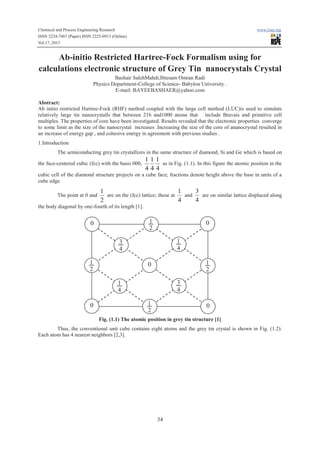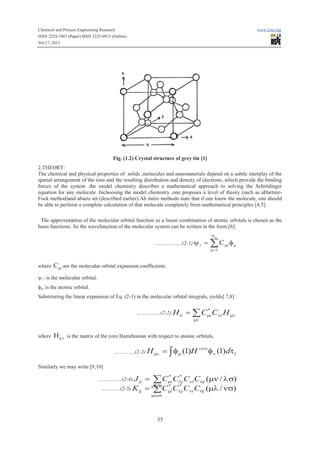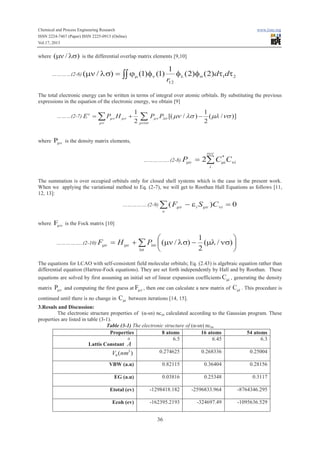Seu SlideShare está sendo baixado. ×

# Ab initio restricted hartree-fock formalism using for calculations electronic structure of grey

Anúncio
Anúncio
Anúncio
Anúncio
Anúncio
Anúncio
Anúncio
Anúncio
Anúncio
Anúncio
AnúncioAnúncio
Anúncio
Carregando em…3
×

1 de 10 Anúncio

# Ab initio restricted hartree-fock formalism using for calculations electronic structure of grey

Anúncio
Anúncio

### Ab initio restricted hartree-fock formalism using for calculations electronic structure of grey

1. 1. Chemical and Process Engineering Research ISSN 2224-7467 (Paper) ISSN 2225-0913 (Online) Vol.17, 2013 www.iiste.org Ab-initio Restricted Hartree-Fock Formalism using for calculations electronic structure of Grey Tin nanocrystals Crystal Bashair SalehMahdi,Ibtesam Omran Radi Physics Department-College of Science- Babylon University . E-mail: BAYEEBASHAER@yahoo.com Abstract: Ab initio restricted Hartree-Fock (RHF) method coupled with the large cell method (LUC)is used to simulate relatively large tin nanocrystalls that between 216 and1000 atoms that include Bravais and primitive cell multiples. The properties of core have been investigated. Results revealed that the electronic properties converge to some limit as the size of the nanocrystal increases .Increasing the size of the core of ananocrystal resulted in an increase of energy gap , and cohesive energy in agreement with previous studies . 1.Introduction The semiconducting grey tin crystallizes in the same structure of diamond, Si and Ge which is based on the face-centered cubic (fcc) with the basis 000, 111 as in Fig. (1.1). In this figure the atomic position in the 444 cubic cell of the diamond structure projects on a cube face; fractions denote height above the base in units of a cube edge. The point at 0 and 3 1 1 are on the (fcc) lattice; those at and are on similar lattice displaced along 4 2 4 the body diagonal by one-fourth of its length . 1 2 0 0 1 4 3 4 1 2 1 2 0 3 4 1 4 0 1 2 0 Fig. (1.1) The atomic position in grey tin structure  Thus, the conventional unit cube contains eight atoms and the grey tin crystal is shown in Fig. (1.2). Each atom has 4 nearest neighbors [2,3]. 34
2. 2. Chemical and Process Engineering Research ISSN 2224-7467 (Paper) ISSN 2225-0913 (Online) Vol.17, 2013 www.iiste.org Fig. (1.2) Crystal structure of grey tin  2.THEORY: The chemical and physical properties of solids ,molecules and nanomaterials depend on a subtle interplay of the spatial arrangement of the ions and the resulting distribution and density of electrons ,which provide the binding forces of the system .the model chemistry describes a mathematical approach to solving the Schrödinger equation for any molecule .Inchoosing the model chemistry ,one proposes a level of theory (such as aHartreeFock method)and abasis set (described earlier).Ab initio methods state that if one know the molecule, one should be able to perform a complete calculation of that molecule completely from mathematical principles [4,5]. The approximation of the molecular orbital function as a linear combination of atomic orbitals is chosen as the basis functions. So the wavefunction of the molecular system can be written in the form : ……………..(2-1) y i No = å C mi f m m =1 where Cmi are the molecular orbital expansion coefficients. ψi : is the molecular orbital. ϕµ :is the atomic orbital. Substituting the linear expansion of Eq. (2-1) in the molecular orbital integrals, yields[ 7,8] : ……………(2-2) H ii where * = å Cmi Cni H mn mn H mn is the matrix of the core Hamiltonian with respect to atomic orbitals, ………….(2-3) H mn = ò f m (1)H core f n (1)dt1 Similarly we may write [9,10] * * = å Cmi Clj Cni Csj (mn / ls) * mlns * …………(2-5) K ij = å Cmi Clj Cni Csj (ml / ns) ……………(2-4) J ij mlns 35
3. 3. Chemical and Process Engineering Research ISSN 2224-7467 (Paper) ISSN 2225-0913 (Online) Vol.17, 2013 www.iiste.org (mn / ls) is the differential overlap matrix elements [9,10] where …………(2-6) (mn / ls) = òò jm (1)fn (1) 1 fl (2)fs (2)dt1dt2 r12 The total electronic energy can be written in terms of integral over atomic orbitals. By substituting the previous expressions in the equation of the electronic energy, we obtain  ………(2-7) E e = where 1 1 å Pmn H mn + 2 mnls Pmn Pls [( mn / ls ) - 2 (ml /ns )] å mn Pmn is the density matrix elements, occ ……………..(2-8) P mn * = 2å Cmi Cni i The summation is over occupied orbitals only for closed shell systems which is the case in the present work. When we applying the variational method to Eq. (2-7), we will get to Roothan Hall Equations as follows [11, 12, 13]: …………….(2-9) å ( Fmn n where - e i S mn )C ni = 0 Fmn is the Fock matrix  ……………..(2-10) Fmn 1 æ ö = H mn + å Pls ç (mn / ls) - (ml / ns) ÷ 2 ls è ø The equations for LCAO with self-consistent field molecular orbitals; Eq. (2.43) is algebraic equation rather than differential equation (Hartree-Fock equations). They are set forth independently by Hall and by Roothan. These equations are solved by first assuming an initial set of linear expansion coefficients Cmi , generating the density matrix Pmn and computing the first guess at Fmn , then one can calculate a new matrix of Cmi . This procedure is continued until there is no change in Cmi between iterations [14, 15]. 3.Resuls and Discussion: The electronic structure properties of (α-sn) ncsn calculated according to the Gaussian program. These properties are listed in table (3-1). Table (3-1) The electronic structure of (α-sn) ncsn Properties 8 atoms 16 atoms 54 atoms 0 6.5 6.45 6.3 Lattis Constant A 0.274625 0.268336 0.25004 V (nm3 ) 0 VBW (a.u) 0.82115 0.36404 0.28156 EG (a.u) 0.03816 0.25348 0.3117 Etotal (ev) -1298418.182 -2596833.964 -8764346.295 Ecoh (ev) -162395.2193 -324697.49 -1095636.529 36
4. 4. Chemical and Process Engineering Research ISSN 2224-7467 (Paper) ISSN 2225-0913 (Online) Vol.17, 2013 www.iiste.org In table (3-1), it is shown that the core part has a converging fluctuating energy gap, valence band width and cohesive energy (absolute value). These fluctuations are related to the geometry of the nanocrystal. The high value of the band gap can be attributed to the approximations involved (the self-interaction of the electrons in the Hartree energy is fully removed) in HF method . The total energy of (α-sn)ncs is calculated from the initial guess of the wavefunction. The new wave function gives a new value of the total energy. The calculated total energy for(α-sn) ncsn as a function of lattice constant for the chosen number of core part atoms (8, 16, and 54) is shown in Fig. (3-1) – (3-3), respectively. The equilibrium lattice constant is at the bottom of the total energy curve. a (nm ) 0.45 0.55 0.65 0.75 0.85 -1298404 -1298406 E tot (eV) -1298408 -1298410 -1298412 -1298414 -1298416 -1298418 Fig.(3-1) Total energy as a function of lattice constant for 8 atom (core) LUC. a nm 0.5 0.55 0.6 0.65 0.7 0.75 0.8 -2596805 -2596810 Etot( eV) -2596815 -2596820 -2596825 -2596830 -2596835 -2596840 Fig.(3-2) Total energy as a function of lattice constant for 16 atom (core) LUC. 37
5. 5. Chemical and Process Engineering Research ISSN 2224-7467 (Paper) ISSN 2225-0913 (Online) Vol.17, 2013 www.iiste.org a nm 0.5 0.55 0.6 0.65 0.7 0.75 0.8 -8764240 -8764260 Etot ( eV) -8764280 -8764300 -8764320 -8764340 -8764360 Fig.(3-3) Total energy as a function of lattice constant for 54 atom (core) LUC. From the above figures, one can see that the total energy decreases with increasing the lattice constant till it reaches the equilibrium lattice constant. After the equilibrium lattice constant, the total energy increases with increasing lattice constant. The behavior of total energy as a function of lattice constant is due to the attraction forces that take place at the large distances between atoms. Decreasing the distance between atoms leads to decreasing the total energy. At shorter distances between atoms, the repulsion forces become dominant and take place to cause the increase of the total energy. The stability of the nanocrystal at the equilibrium lattice constant is due to the equality of attraction and repulsion forces at this point . On the other hand, it is shown that the total energy for core part decreases with increasing the number of atoms per LUC as shown in fig. (3-4). On the scale shown in this figure, the size dependence of the energy is linear. The total energy has a high sensitivity to the potential energy of the crystal. num ber of atom 0 5 10 15 20 25 30 35 40 45 50 55 60 0 -1400000 Etot (eV) -2800000 -4200000 -5600000 -7000000 -8400000 -9800000 Fig. (3-4) Total energy as a function of number of atom for all size of core LUC. Fig.(3-5) shows the relationship between the lattice constant and the number of atoms for all studied LUC sizes of (α-sn)ncsn core. The lattice constants show decreasing values from 0.65 nm for 8 atoms to 0.632 nm for 54 atoms LUC 38
6. 6. Chemical and Process Engineering Research ISSN 2224-7467 (Paper) ISSN 2225-0913 (Online) Vol.17, 2013 www.iiste.org 0.655 0.645 0.64 0.635 lattace constant (nm) 0.65 0.63 0.625 60 50 40 30 20 10 0 num ber of atom Fig. (3-5) Lattice constant as a function of number of atoms for (α-sn)ncsn LUC. The lattice constant of the LUC is varied until we determine the minimum energy for each cell. These minima are plotted in Fig. (3-5). By lattice constant which is inside the core of the nanocrystal. The LUC calculations are far more stable than molecular dynamics calculations of nanoclusters that include the surface reconstruction optimization. No optimization is needed for the core part except the aforementioned lattice constant optimization. num ber of atom 0 10 20 30 40 50 60 0 Eco (eV) -200000 -400000 -600000 -800000 -1000000 -1200000 Fig. (3-6). Cohesive energy as a function of number of atom for α-sn (ncsn) LUC. In Fig. (3-6), the absolute value of the cohesive energy increases with increasing the number of atoms per LUC, because the small (α-sn)particle revealed more suspension bond and a high activation energy. The atoms on the particle’s surface reconstructed to a more stable structure. The calculated cohesive energy is directly proportional to the value of the total energy. The correct calculated value of the total energy gives a correct value of the cohesive energy. This result is in good agreement with reports on cohesive energy (α-sn) nanoparticles increases with an increasing particle size [17, 18]. One of the most important electronic properties of solid is the energy gap. The energy gap is the difference between the Lowest Unoccupied Molecular Orbital (LUMO) and the Highest Occupied Molecular Orbital (HOMO) . The energy gap in (eV) as a function of lattice constant for all size of (α-sn)ncsn core is shown in Fig.(37). 39
7. 7. Chemical and Process Engineering Research ISSN 2224-7467 (Paper) ISSN 2225-0913 (Online) Vol.17, 2013 www.iiste.org 2.72 energy gab (eV) 2.68 2.64 2.6 2.56 2.52 2.48 0.625 0.63 0.635 0.64 0.645 0.65 0.655 lattace constant (nm ) Fig.(3-7) Energy gap as a function of lattice constant for(α-sn)ncsn LUC. In Fig. (3-7), the energy gap is shown to decrease with increasing lattice constant. This result is in good agreement with reference  when they studied Ge ncs for different size. The energy gap in (eV) as a function of number of atoms for all sizes of core is (α-sn)ncsn shown in Fig.(3-8). 2.75 energy gab (eV) 2.7 2.65 2.6 2.55 2.5 2.45 0 5 10 15 20 25 30 35 40 45 50 55 60 num ber of atom Fig.(3-8) Energy gap as a function of number of atom for(α-sn) ncsn LUC. In Fig.(3-8), the energy gap is shown to increase with increasing the number of atoms. After that the energy gap has nearly stable value with some fluctuations. This figure shows a converging behavior when the number of core atoms increases. This convergence is associated with fluctuations depending on the geometry of the nanocrystal core . At the convergence plateau Bravais cubic lattices seem to have high energy gaps. This is a clear indication of geometry effects on electronic structure of nanocrystals. Energy gap is inversely proportional to the surface area of core atoms. Parallelepiped cells have less gap values than cubic ones. This discrimination between the electronic properties is also applicable to cohesive and valence band energies. These differences diminish as the nanocrystal grows up in size. The valence band width for the core of different size is shown in Fig. (3-9), and the valence band width as a function of lattice constant is shown in Fig. (3-10). In Fig. (3-9) the valence band width is shown to increase with increasing number of atom per LUC, because of the geometry effects on electronic structure of nanocrystals. At the convergence plateau Bravais cubic lattices seem to have higher valence band width  We note from the above values that the first and third values (Bravais) are higher than the surrounding second and fourth (primitive) values 40
8. 8. Chemical and Process Engineering Research ISSN 2224-7467 (Paper) ISSN 2225-0913 (Online) Vol.17, 2013 www.iiste.org valance band wedth (eV) 12 10 8 6 4 2 0 0 5 10 15 20 25 30 35 40 45 50 55 60 num ber of atom .Fig. (3-9). The valence band width as a function of number of atoms for (α-sn) ncsn LUC. 10 valance band wedth (eV) 9 8 7 6 5 4 3 2 1 0 0.625 0.63 0.635 0.64 0.645 0.65 0.655 lattce constant (nm ) Fig. (3-10) Valence band width as a function of lattice constant for all size of Si nc s LUC. In Fig.(3-10) one can notice that when the lattice constant decreases the width of the valence band increases, as it was reported for Grey tin crystals [16, 22]. References 1.C. Kittle, "Introduction to solid state physics", John Wiely & Sons, (1996). 2.H.P. Myers, "Introductory solid state physics", Taylor & Francis, (1990). 3.W.J. Moore, "Physical chemistry", Longmans, Green and Co, (1957). 4. C. Lamberti, " Characterization of Semiconductor Heterostructures and Nanostructures", Elsevier Publications , (2008) 5.H. Fehske, R. Schneider, and A. Weibe , " Computational Many Particle Physics", Springer, Berlin Heidelberg, (2013). 6.Simons, "An introduction to theoretical chemistry", Cambridge University Press, (2003). 7.P. R. Taylor, ”Quantum Theory of Molecular Electronic Structure”, San Diego Supercomputer Center and Department of Chemistry and Biochemistry, University of California, (1997), taylor@sdsc.eduhtt 8.G. A. Gallup, "Valence Bond Methods: Theory and Applications", Cambridge University Press ", (2000). 41
9. 9. Chemical and Process Engineering Research ISSN 2224-7467 (Paper) ISSN 2225-0913 (Online) Vol.17, 2013 www.iiste.org 9.J.A. Pople and D.L. Beveridge, “Approximate Molecular Orbital Theory”, Mc Graw-Hill, (1970). 1o.H. Sodergren ,"Calculations of Optical Absorption in Low-Bandgap Polymers and Molecules Using TimeDependent Quantum Chemical Methods , MSc. Thesis, (2004). 11.W. Hehre, L. Random, P. Schleyer, and J. Pople, "Ab-initio Molecular Orbital Theory ", John Wiley and Sons (1986). 12.W. Demtroder, "Molecular Physics", Wiley-Vch ,(2005). 13.M. A. Abdulsattar, Physica E 41(1679), (2009). 14.J. A. Pople, "Quantum Chemical Models", Northwestern University, (1998). 15. M. G. Merdan, "Self-Consistent Field Calculations for the Effect of Pressure and Temperature on Some Properties of Grey Tin Crystal", M.Sc Thesis, University of Babylon, (2005). 16. Mohammad G. Merdan, "Self-Consistent Field Calculations for the Effect of Pressure and Temperature on Some Properties of Grey Tin Crystal", M.Sc Thesis, (2005). 17. K.C. Fang, C.I.Weng, and S.P. Ju, Nanotechnology, 7(3909), (2006). 18. I. Kwon, R. Biswas, C.Z. Wang, k. m. Ho, and C.M. Soukoulis, Phy. Rev. B, 49(11), (1993). 19. M. Heidari Saani, M. Kargarian, and A. Ranjbar, Phys. Rev. B 76( 035417), (2007). 20. P. Alfaro, A. Miranda, A.E. Ramos, and M.C. Irisson, Brazilian Journal of Physics, 36 (2A), (2012). 21. S. M. Sze and K. K. Ng, "Physics of semiconductor devices", 3rd edition, Wiley, (2007). 22. John N. Murrell, Sydney F.A. Kettle and J.M. Tedder, "The Chemical Bond", Translated to Arabic by M.N. Al-Zakum, Al-Basrah University (1983). 42